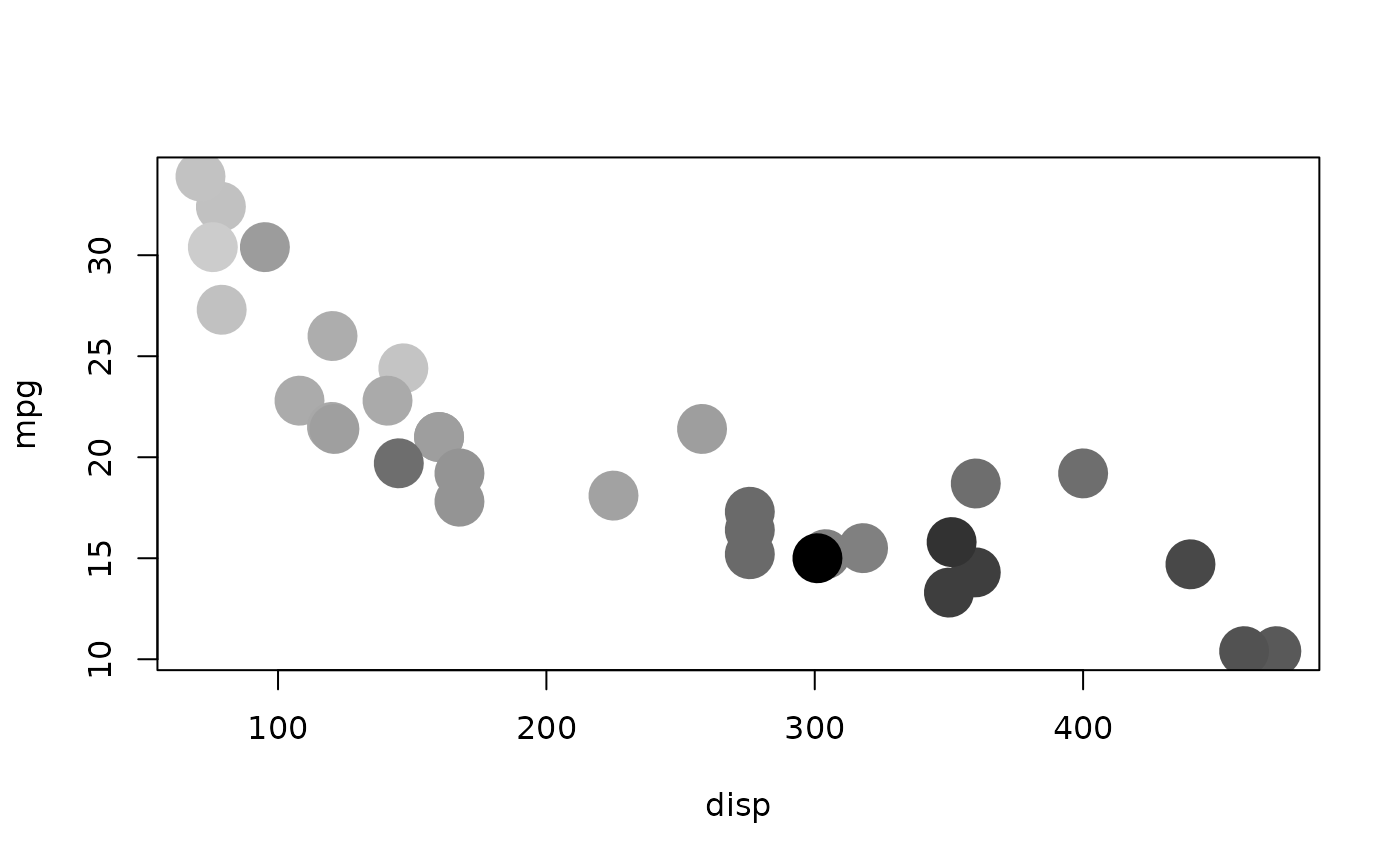Continuous scale

cscale(x, palette, na.value = NA_real_, trans = identity_trans())

## Arguments

x vector of continuous values to scale palette to use. Built in palettes: area_pal, brewer_pal, dichromat_pal, div_gradient_pal, gradient_n_pal, grey_pal, hue_pal, identity_pal, linetype_pal, manual_pal, rescale_pal, seq_gradient_pal, shape_pal, viridis_pal value to use for missing values transformation object describing the how to transform the raw data prior to scaling. Defaults to the identity transformation which leaves the data unchanged. Built in transformations: asn_trans, atanh_trans, boxcox_trans, date_trans, exp_trans, hms_trans, identity_trans, log_trans, log10_trans, log1p_trans, log2_trans, logit_trans, modulus_trans, probability_trans, probit_trans, pseudo_log_trans, reciprocal_trans, reverse_trans, sqrt_trans, time_trans, yj_trans .

## Examples

with(mtcars, plot(disp, mpg, cex = cscale(hp, rescale_pal())))with(mtcars, plot(disp, mpg, cex = cscale(hp, rescale_pal(),
trans = sqrt_trans())))with(mtcars, plot(disp, mpg, cex = cscale(hp, area_pal())))with(mtcars, plot(disp, mpg, pch = 20, cex = 5,
col = cscale(hp, seq_gradient_pal("grey80", "black"))))